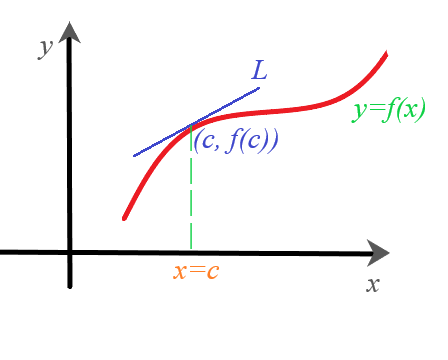# Find: a. The slope of the curve at the given point P, b. An equation of the tangent line at P....

## Question:

Find:

a. The slope of the curve at the given point {eq}P, {/eq}

b. An equation of the tangent line at {eq}P. {/eq}

{eq}y=-3-6x^2; \ P(-5,-153) {/eq}

## Slope and Tangent Line

The value of the derivative at a given point on the function is the instantaneous rate of change of a function. The derivative can also be defined as the slope of the tangent line to a curve. The tangent line to the curve {eq}y=f(x) {/eq} at {eq}x=c {/eq} is shown below.The slope of the tangent line L is the value of {eq}\displaystyle \frac{dy}{dx} {/eq} at {eq}(c,f(c)). {/eq} To find the equation of the tangent line we can use the point-slope equation of the straight line.

We are given a curve {eq}y=-3-6x^2 {/eq} and a point on the curve {eq}P(-5,-153). {/eq}

a. We are asked to find the slope of the given curve at {eq}P {/eq}. To do this, we will take its derivative derivative and substitute the value of {eq}x {/eq} from the point {eq}P. {/eq} into the derivative.

\begin{align*} \frac{dy}{dx} &=\frac{d}{dx}\left ( -3-6x^2 \right )\\ &=\frac{d}{dx}\left ( -3 \right )+\frac{d}{dx}\left ( -6x^2 \right )\\ &=0-6*2x\\ &=-12x\\ \frac{dy}{dx}\bigg|_{(-5,-153)} &=-12*-5\\ &=60. \end{align*}

Hence the slope is {eq}60. {/eq}

b. We are also asked to find the equation of the tangent line at {eq}P(-5,-153) {/eq}. We already know the slope of the line and we are given a point. To find the equation of the line, we will use the slope-point form of the line, {eq}y - y_0 = m(x - x_0) {/eq}.

\begin{align*} y-(-153) &=\frac{dy}{dx}\bigg|_{(-5,-153)}\left ( x-(-5) \right )\\ y+153&=60(x+5)\\ y &=60x+300\\ y &=60x+300-153\\ y &= 60x + 147 \end{align*}

Therefore, {eq}{\color{Blue} {y=60x+147}} {/eq} is the equation of the tangent line.